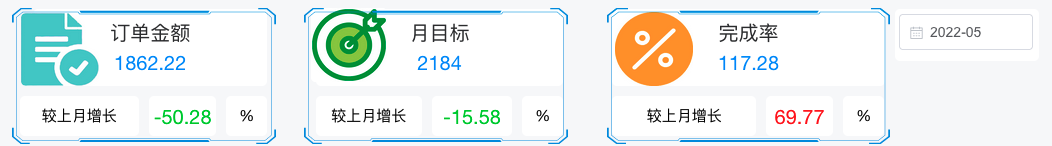# 通过 SQL 实现在指标卡视图中展示同比和环比数值

0 评论 / 0 点赞 / 202 阅读 最后更新: 2022-09-08 作者: 飞致云 总字数: 2423

# 1 场景需求

DataEase 可以在柱状图中计算同环比，目前还不支持直接用指标卡来计算同环比展示下图的效果：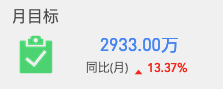# 2 实现方法

## 2.1 准备演示数据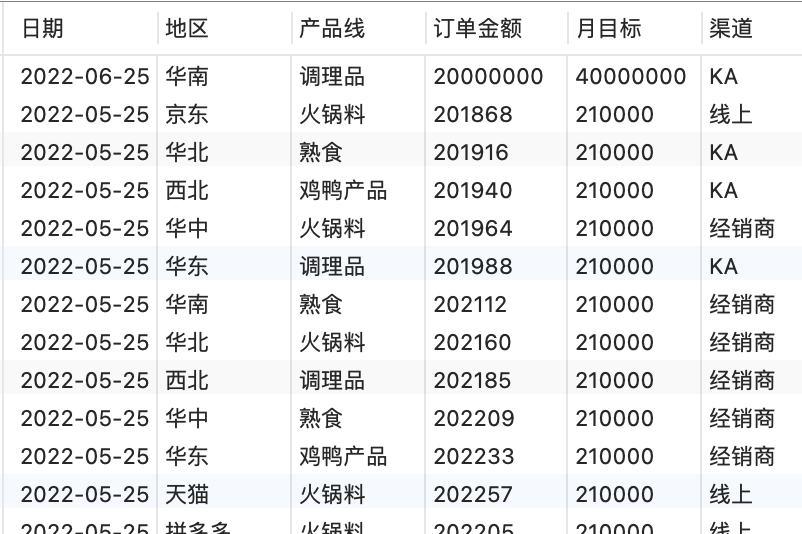## 2.2 SQL计算过程

### 2.2.1 聚合日期

``````select DATE_FORMAT(日期,'%Y-%m') as date, SUM(月目标) as 月目标 from xiaoshou GROUP BY date
``````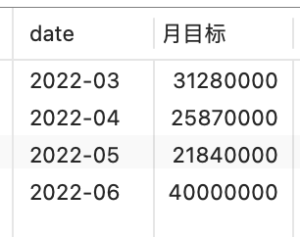### 2.2.2 增加字段参考计算

``````select DATE_FORMAT(DATE_ADD(日期,INTERVAL 1 MONTH),'%Y-%m') as date, SUM(月目标) as 月目标 from xiaoshou GROUP BY date
``````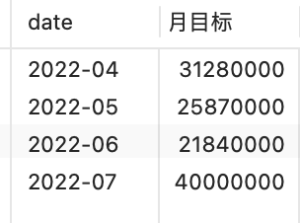### 2.2.3 左连接整合数据

``````select CONCAT(t.date,'-01') tdate ,t.月目标 t月目标 ,y.月目标 y月目标

from

(select DATE_FORMAT(日期,'%Y-%m') as date, SUM(月目标) as 月目标 from xiaoshou GROUP BY date) t

left JOIN

(select DATE_FORMAT(DATE_ADD(日期,INTERVAL 1 MONTH),'%Y-%m') as date, SUM(月目标) as 月目标 from xiaoshou GROUP BY date) y

on t.date = y.date
``````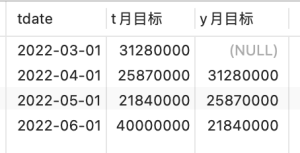### 2.2.4 计算环比

``````select CONCAT(t.date,'-01') tdate ,t.月目标 t月目标 , y.月目标 y月目标 ,

case

when y.月目标 is null or y.月目标 = 0 then 0.00

else round((t.月目标 - y.月目标) / y.月目标 * 100, 2)

end ratio

from

(select DATE_FORMAT(日期,'%Y-%m') as date, SUM(月目标) as 月目标 from xiaoshou GROUP BY date) t

left JOIN

(select DATE_FORMAT(DATE_ADD(日期,INTERVAL 1 MONTH),'%Y-%m') as date, SUM(月目标) as 月目标 from xiaoshou GROUP BY date) y

on t.date = y.date
``````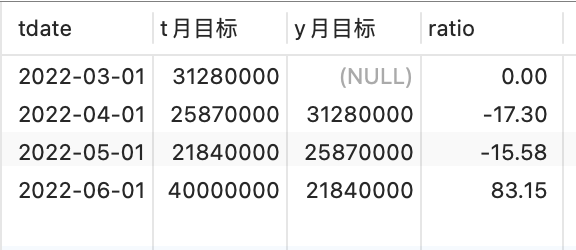# 3 深度应用

``````select CONCAT(t.date,'-01') tdate ,t.月目标 t月目标 , y.月目标 y月目标 , t.月订单金额 t月订单金额 , y.月订单金额 y月订单金额 , t.完成率 t完成率 , y.完成率 y完成率,

case

when y.月目标 is null or y.月目标 = 0 then 0.00

else round((t.月目标 - y.月目标) / y.月目标 * 100, 2)

end 月目标ratio ,

case

when y.月订单金额 is null or y.月订单金额 = 0 then 0.00

else round((t.月订单金额 - y.月订单金额) / y.月订单金额 * 100, 2)

end 月订单ratio ,

case

when y.完成率 is null or y.完成率 = 0 then 0.00

else round((t.完成率 - y.完成率) / y.完成率 * 100, 2)

end 完成率ratio

from

(select DATE_FORMAT(日期,'%Y-%m') as date, SUM(月目标) as 月目标, sum(订单金额) as 月订单金额 ,ROUND(SUM(月目标)/sum(订单金额)*100,2) as 完成率 from xiaoshou GROUP BY date) t

left JOIN

(select DATE_FORMAT(DATE_ADD(日期,INTERVAL 1 MONTH),'%Y-%m') as date, SUM(月目标) as 月目标 ,sum(订单金额) as 月订单金额 ,ROUND(SUM(月目标)/sum(订单金额)*100,2) as 完成率 from xiaoshou GROUP BY date) y

on t.date = y.date
``````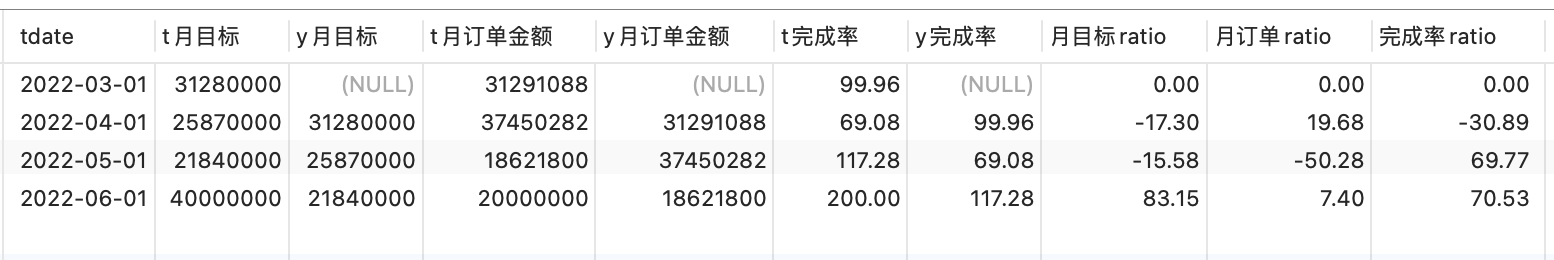“t 月目标” “y 月目标” ：分别代表当月目标和上月目标；

“t 月订单金额” “y 月订单金额” ：分别代表当月订单和上月订单；

“t 完成率” “y 完成率” ：分别代表当月完成率和上月完成率；

“月目标 ratio” “月订单 ratio” “完成率 ratio”：分别代表月目标环比、月订单环比、完成率环比。

# 4 DataEase 中展示效果

“订单金额”、“月目标”、“完成率”，三个指标卡视图下方的 “环比（月）” 关联我们最终制作的数据集对应的”月订单 ratio“、”月目标 ratio“、”完成率 ratio“；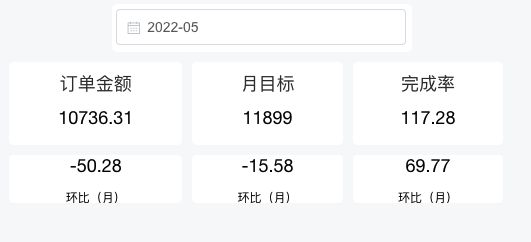# 5 最终美化效果